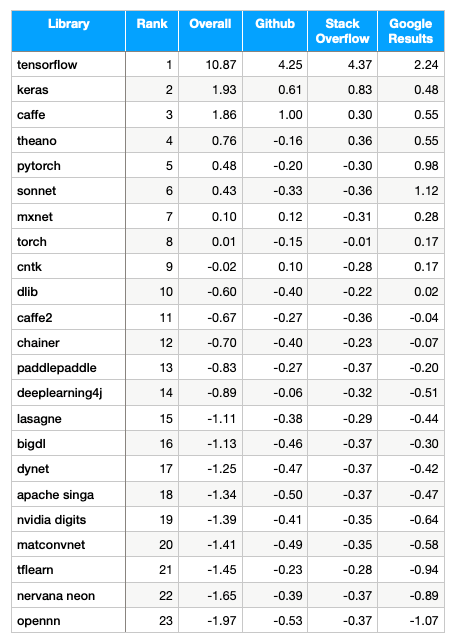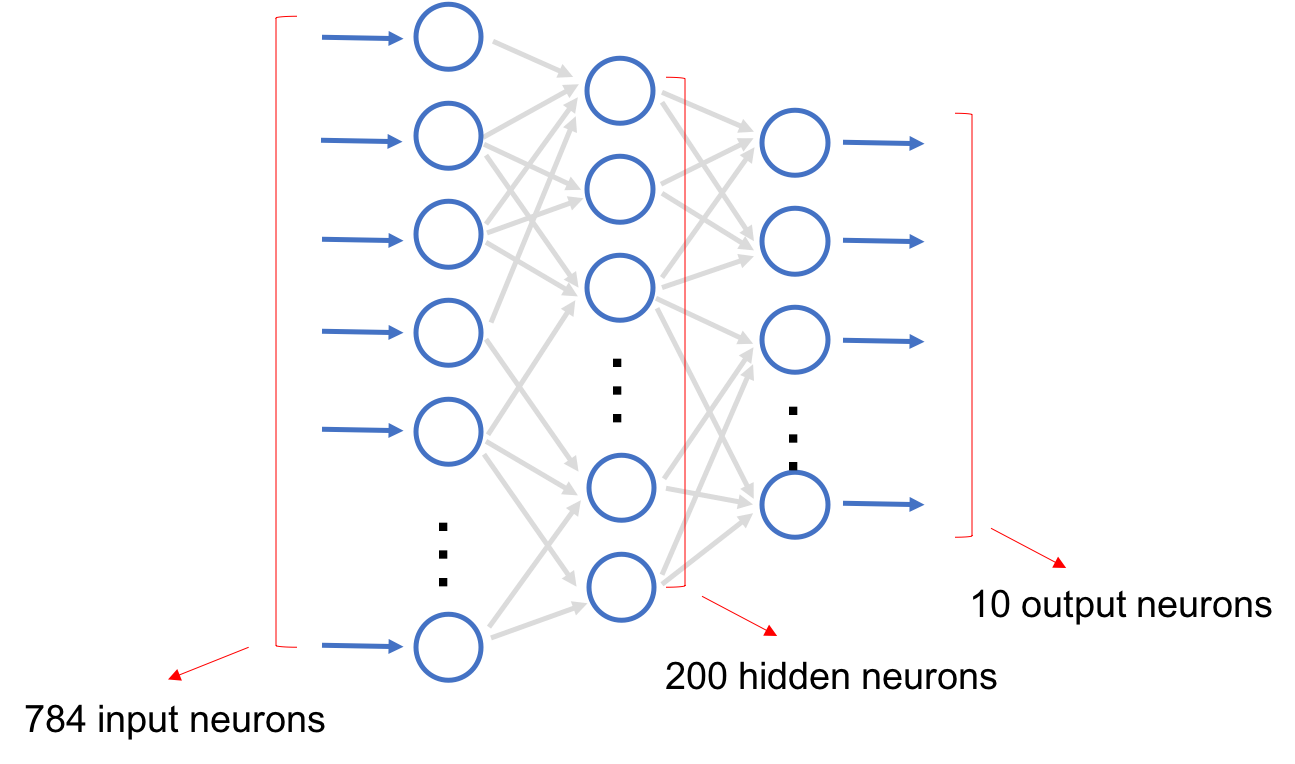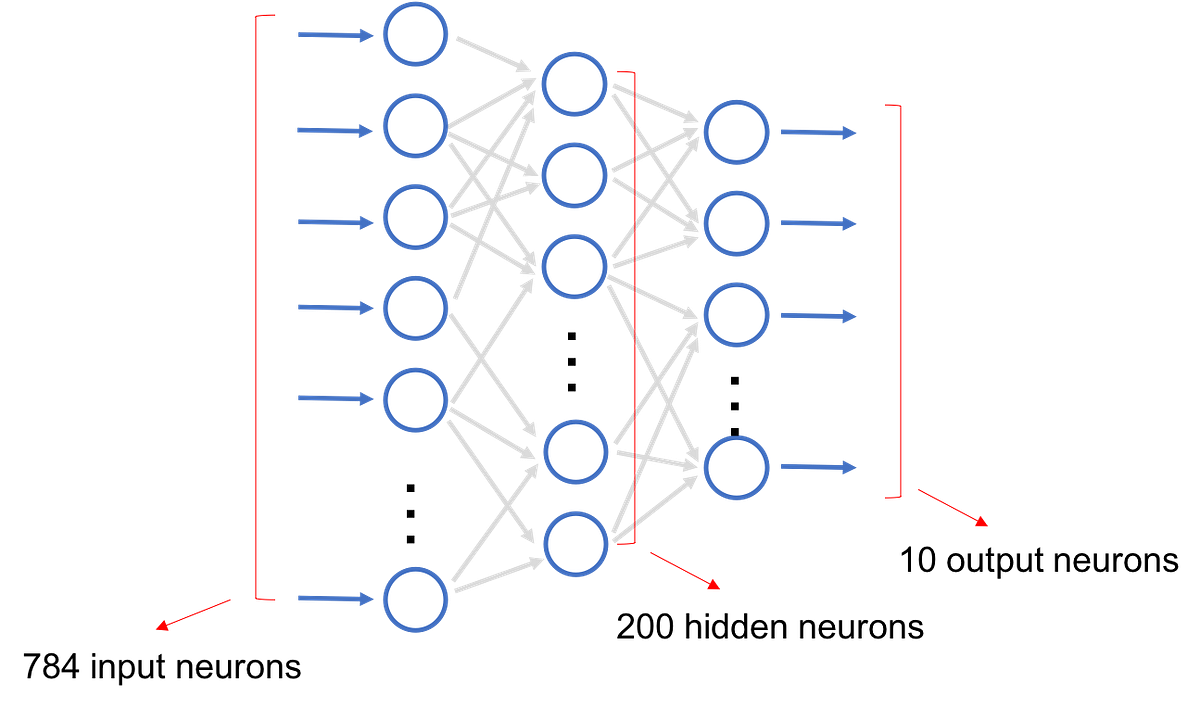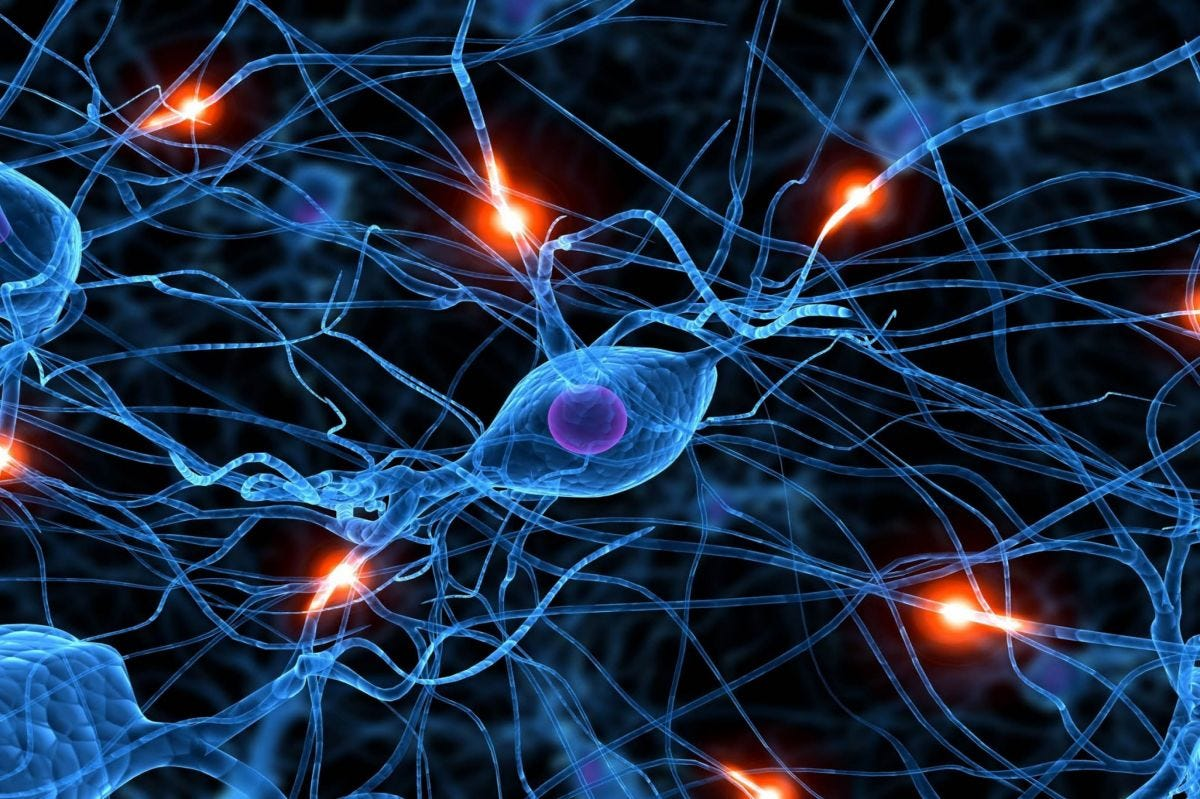1596078221

# Building simple artificial neural networks with TensorFlow, Keras, PyTorch and MXNet/Gluon

A few weeks ago I went through the steps of building a very simple neural network and implemented it from scratch in Go. However there are many deep learning frameworks that are already available, so doing it from scratch isn’t normally what you’ll do if you want to use deep learning as a tool to solve problems.

The question is with the many that deep learning frameworks, which one should I use? There are many ways to compare deep learning frameworks. Here’s a relatively recent (September 2017) ranking by Data Incubator that gives an interesting popularity ranking based on their Github, Stack Overflow and Google search results scores.From the results, it’s quite clear that TensorFlow is easily the most popular framework and with Keras now being a part of TensorFlow itself, things won’t change much in the near future. Also, almost all popular deep learning frameworks have Python APIs, so a combination of TensorFlow/Keras with Python seems the way to go.

Nonetheless, I was curious about some of the other frameworks and so I began a mini-journey to write the same (or almost the same) simple artificial neural network I did in a few of these frameworks for comparison.

As a quick refresher, the neural network I created was a simple feed-forward neural network, also commonly called a multi-level perceptron (MLP). Using this simple MLP, I took the MNIST dataset of 60,000 handwritten digits and trained the neural network with it. After that I used the test dataset of 10,000 handwritten digits to test the accuracy of the neural network.Our simple artificial neural network

The neural network had 3 layers, the first (input) layer has 784 neurons (28 x 28 pixels), the second (hidden) layer has 200 neurons and the final (output) layer has 10 neurons. I used the sigmoid function as the activation function, and also mean square error as the loss function. Finally, I used 0.1 as the learning rate and didn’t use any bias neurons at all.

All the implementations below follow the same generic steps:

1. Set up the parameters and load the datasets (most frameworks have a means to load standard datasets like MNIST)
2. Define the neural network by creating a `mlp` function that creates and returns the neural network
3. Define the `train` function
4. Define the `predict` function
5. Create a main that allows the user to first train using the training dataset (60,000 images) then predict using the test dataset (10,000 images)

This handwriting recognition of digits with the MNIST dataset is so often used in deep learning tutorials it’s almost the ‘hello world’ of writing deep learning programs. As a disclaimer though, the implementations you see below are not optimized in any way and are not the definitive way of doing it. In fact there are many other more optimal ways of doing it, these are just a few.

Now let’s start and see how to implement this in TensorFlow first.

#python #keras #pytorch #tensorflow #machine-learning

## Buddha Community1596078221

## Building simple artificial neural networks with TensorFlow, Keras, PyTorch and MXNet/Gluon

A few weeks ago I went through the steps of building a very simple neural network and implemented it from scratch in Go. However there are many deep learning frameworks that are already available, so doing it from scratch isn’t normally what you’ll do if you want to use deep learning as a tool to solve problems.

The question is with the many that deep learning frameworks, which one should I use? There are many ways to compare deep learning frameworks. Here’s a relatively recent (September 2017) ranking by Data Incubator that gives an interesting popularity ranking based on their Github, Stack Overflow and Google search results scores.From the results, it’s quite clear that TensorFlow is easily the most popular framework and with Keras now being a part of TensorFlow itself, things won’t change much in the near future. Also, almost all popular deep learning frameworks have Python APIs, so a combination of TensorFlow/Keras with Python seems the way to go.

Nonetheless, I was curious about some of the other frameworks and so I began a mini-journey to write the same (or almost the same) simple artificial neural network I did in a few of these frameworks for comparison.

As a quick refresher, the neural network I created was a simple feed-forward neural network, also commonly called a multi-level perceptron (MLP). Using this simple MLP, I took the MNIST dataset of 60,000 handwritten digits and trained the neural network with it. After that I used the test dataset of 10,000 handwritten digits to test the accuracy of the neural network.Our simple artificial neural network

The neural network had 3 layers, the first (input) layer has 784 neurons (28 x 28 pixels), the second (hidden) layer has 200 neurons and the final (output) layer has 10 neurons. I used the sigmoid function as the activation function, and also mean square error as the loss function. Finally, I used 0.1 as the learning rate and didn’t use any bias neurons at all.

All the implementations below follow the same generic steps:

1. Set up the parameters and load the datasets (most frameworks have a means to load standard datasets like MNIST)
2. Define the neural network by creating a `mlp` function that creates and returns the neural network
3. Define the `train` function
4. Define the `predict` function
5. Create a main that allows the user to first train using the training dataset (60,000 images) then predict using the test dataset (10,000 images)

This handwriting recognition of digits with the MNIST dataset is so often used in deep learning tutorials it’s almost the ‘hello world’ of writing deep learning programs. As a disclaimer though, the implementations you see below are not optimized in any way and are not the definitive way of doing it. In fact there are many other more optimal ways of doing it, these are just a few.

Now let’s start and see how to implement this in TensorFlow first.

#python #keras #pytorch #tensorflow #machine-learning1590626367

## Artificial Neural Networks using Keras and TensorFlow in Python

### What you’ll learn

Get a solid understanding of Artificial Neural Networks (ANN) and Deep Learning
Understand the business scenarios where Artificial Neural Networks (ANN) is applicable
Building a Artificial Neural Networks (ANN) in Python
Use Artificial Neural Networks (ANN) to make predictions
Learn usage of Keras and Tensorflow libraries
Use Pandas DataFrames to manipulate data and make statistical computations.

Requirements

• Students will need to install Python and Anaconda software but we have a separate lecture to help you install the sameStudents will need to install Python and Anaconda software but we have a separate lecture to help you install the same

Description

You’re looking for a complete Artificial Neural Network (ANN) course that teaches you everything you need to create a Neural Network model in Python, right?

You’ve found the right Neural Networks course!

After completing this course you will be able to:

• Identify the business problem which can be solved using Neural network Models.
• Have a clear understanding of Advanced Neural network concepts such as Gradient Descent, forward and Backward Propagation etc.
• Create Neural network models in Python using Keras and Tensorflow libraries and analyze their results.
• Confidently practice, discuss and understand Deep Learning concepts

Verifiable Certificate of Completion is presented to all students who undertake this Neural networks course.

If you are a business Analyst or an executive, or a student who wants to learn and apply Deep learning in Real world problems of business, this course will give you a solid base for that by teaching you some of the most advanced concepts of Neural networks and their implementation in Python without getting too Mathematical.

Why should you choose this course?

This course covers all the steps that one should take to create a predictive model using Neural Networks.

Most courses only focus on teaching how to run the analysis but we believe that having a strong theoretical understanding of the concepts enables us to create a good model . And after running the analysis, one should be able to judge how good the model is and interpret the results to actually be able to help the business.

What makes us qualified to teach you?

The course is taught by Abhishek and Pukhraj. As managers in Global Analytics Consulting firm, we have helped businesses solve their business problem using Deep learning techniques and we have used our experience to include the practical aspects of data analysis in this course

We are also the creators of some of the most popular online courses - with over 250,000 enrollments and thousands of 5-star reviews like these ones:

This is very good, i love the fact the all explanation given can be understood by a layman - Joshua

Thank you Author for this wonderful course. You are the best and this course is worth any price. - Daisy

Our Promise

Teaching our students is our job and we are committed to it. If you have any questions about the course content, practice sheet or anything related to any topic, you can always post a question in the course or send us a direct message.

With each lecture, there are class notes attached for you to follow along. You can also take practice test to check your understanding of concepts. There is a final practical assignment for you to practically implement your learning.

What is covered in this course?

This course teaches you all the steps of creating a Neural network based model i.e. a Deep Learning model, to solve business problems.

Below are the course contents of this course on ANN:

• Part 1 - Python basics
• This part gets you started with Python.
• This part will help you set up the python and Jupyter environment on your system and it’ll teach you how to perform some basic operations in Python. We will understand the importance of different libraries such as Numpy, Pandas & Seaborn.
• Part 2 - Theoretical Concepts
• This part will give you a solid understanding of concepts involved in Neural Networks.
• In this section you will learn about the single cells or Perceptrons and how Perceptrons are stacked to create a network architecture. Once architecture is set, we understand the Gradient descent algorithm to find the minima of a function and learn how this is used to optimize our network model.
• Part 3 - Creating Regression and Classification ANN model in Python
• In this part you will learn how to create ANN models in Python.
• We will start this section by creating an ANN model using Sequential API to solve a classification problem. We learn how to define network architecture, configure the model and train the model. Then we evaluate the performance of our trained model and use it to predict on new data. We also solve a regression problem in which we try to predict house prices in a location. We will also cover how to create complex ANN architectures using functional API. Lastly we learn how to save and restore models.
• We also understand the importance of libraries such as Keras and TensorFlow in this part.
• Part 4 - Data Preprocessing
• In this part you will learn what actions you need to take to prepare Data for the analysis, these steps are very important for creating a meaningful.
• In this section, we will start with the basic theory of decision tree then we cover data pre-processing topics like missing value imputation, variable transformation and Test-Train split.
• Part 5 - Classic ML technique - Linear Regression
• This section starts with simple linear regression and then covers multiple linear regression.
• We have covered the basic theory behind each concept without getting too mathematical about it so that you
• understand where the concept is coming from and how it is important. But even if you don’t understand
• it, it will be okay as long as you learn how to run and interpret the result as taught in the practical lectures.
• We also look at how to quantify models accuracy, what is the meaning of F statistic, how categorical variables in the independent variables dataset are interpreted in the results and how do we finally interpret the result to find out the answer to a business problem.

By the end of this course, your confidence in creating a Neural Network model in Python will soar. You’ll have a thorough understanding of how to use ANN to create predictive models and solve business problems.

42,611 students enrolled

Go ahead and click the enroll button, and I’ll see you in lesson 1!

#tensorflow #python #keras #neural networks1617764209

## Building an Artificial Neural Network in Tensorflow2.0

In this notebook, we will see how to implement an Artificial Neural Network using Tensorflow 2.0. We will be using Fashion MNIST dataset directly importing it from Tensorflow datasets

## Importing libraries

``````import numpy as np
import datetime
import tensorflow as tf
from tensorflow.keras.datasets import fashion_mnist
tf.__version__
'2.3.0'
``````

#deep-learning #tensorflow #artificial-neural-network #keras #fashion-mnist1623135499

## No Code introduction to Neural Networks

### The simple architecture explained

Neural networks have been around for a long time, being developed in the 1960s as a way to simulate neural activity for the development of artificial intelligence systems. However, since then they have developed into a useful analytical tool often used in replace of, or in conjunction with, standard statistical models such as regression or classification as they can be used to predict or more a specific output. The main difference, and advantage, in this regard is that neural networks make no initial assumptions as to the form of the relationship or distribution that underlies the data, meaning they can be more flexible and capture non-standard and non-linear relationships between input and output variables, making them incredibly valuable in todays data rich environment.

In this sense, their use has took over the past decade or so, with the fall in costs and increase in ability of general computing power, the rise of large datasets allowing these models to be trained, and the development of frameworks such as TensforFlow and Keras that have allowed people with sufficient hardware (in some cases this is no longer even an requirement through cloud computing), the correct data and an understanding of a given coding language to implement them. This article therefore seeks to be provide a no code introduction to their architecture and how they work so that their implementation and benefits can be better understood.

Firstly, the way these models work is that there is an input layer, one or more hidden layers and an output layer, each of which are connected by layers of synaptic weights¹. The input layer (X) is used to take in scaled values of the input, usually within a standardised range of 0–1. The hidden layers (Z) are then used to define the relationship between the input and output using weights and activation functions. The output layer (Y) then transforms the results from the hidden layers into the predicted values, often also scaled to be within 0–1. The synaptic weights (W) connecting these layers are used in model training to determine the weights assigned to each input and prediction in order to get the best model fit. Visually, this is represented as:

#machine-learning #python #neural-networks #tensorflow #neural-network-algorithm #no code introduction to neural networks1597632480

## Build your own Neural Network for CIFAR-10 using PyTorch

In 6 simple steps

Neural network seems like a black box to many of us. What happens inside it, how does it happen, how to build your own neural network to classify the images in datasets like MNIST, CIFAR-10 etc. are the questions that keep popping up. Let’s try to understand a Neural Network in brief and jump towards building it for CIFAR-10 dataset. By the end of this article you will have answers to :

1. What are neural networks?
2. How to build a neural network model for cifar-10 dataset by using PyTorch?### What are neural networks?

Neural networks(NN) are inspired by the human brain. A neuron in a human brain, individually is at rest until it collects signals from others through a structure called dendrites, when the excitation that it receives is sufficiently high, the neuron is fired up(gets activated) and it passes on the information. Artificial neural networks(ANN) are made up of interconnected model/artificial neurons(known as perceptron) that take many weighted inputs , add them up and pass it through a non-linearity to produce an output. Sounds simple!

#neural-networks #machine-learning #pytorch #cifar-10 #neural networks